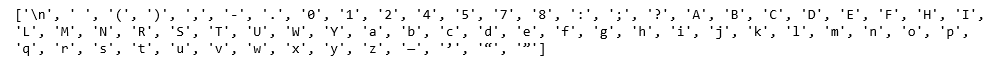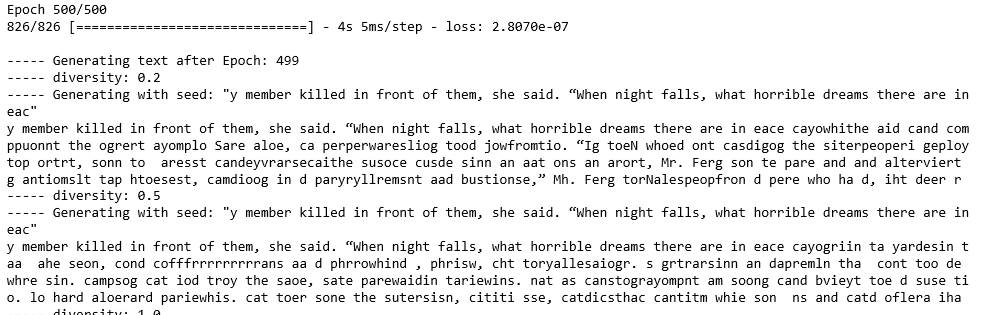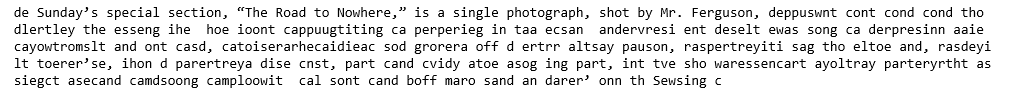Text Generation using Recurrent Long Short Term Memory Network

• Difficulty Level : Medium
• Last Updated : 25 Jun, 2021

This article will demonstrate how to build a Text Generator by building a Recurrent Long Short Term Memory Network. The conceptual procedure of training the network is to first feed the network a mapping of each character present in the text on which the network is training to a unique number. Each character is then hot-encoded into a vector which is the required format for the network.
The data for the described procedure was downloaded from Kaggle. This dataset contains the articles published in the New York Times from April 2017 to April 2018. separated according to the month of publication. The dataset is in the form of .csv file which contains the url of the published article along with other details. Any one random url was chosen for the training process and then on visiting this url, the text was copied into a text file and this text file was used for the training process.
Step 1: Importing the required libraries

Python3

 from __future__ import absolute_import, division,                       print_function, unicode_literals import numpy as npimport tensorflow as tf from keras.models import Sequentialfrom keras.layers import Dense, Activationfrom keras.layers import LSTM from keras.optimizers import RMSprop from keras.callbacks import LambdaCallbackfrom keras.callbacks import ModelCheckpointfrom keras.callbacks import ReduceLROnPlateauimport randomimport sys

Python3

 # Changing the working location to the location of the text filecd C:\Users\Dev\Desktop\Kaggle\New York Times # Reading the text file into a stringwith open('article1.txt', 'r') as file:    text = file.read() # A preview of the text file   print(text)Step 3: Creating a mapping from each unique character in the text to a unique number

Python3

 # Storing all the unique characters present in the textvocabulary = sorted(list(set(text))) # Creating dictionaries to map each character to an indexchar_to_indices = dict((c, i) for i, c in enumerate(vocabulary))indices_to_char = dict((i, c) for i, c in enumerate(vocabulary)) print(vocabulary)Step 4: Pre-processing the data

Python3

 # Dividing the text into subsequences of length max_length# So that at each time step the next max_length characters# are fed into the networkmax_length = 100steps = 5sentences = []next_chars = []for i in range(0, len(text) - max_length, steps):    sentences.append(text[i: i + max_length])    next_chars.append(text[i + max_length])     # Hot encoding each character into a boolean vectorX = np.zeros((len(sentences), max_length, len(vocabulary)), dtype = np.bool)y = np.zeros((len(sentences), len(vocabulary)), dtype = np.bool)for i, sentence in enumerate(sentences):    for t, char in enumerate(sentence):        X[i, t, char_to_indices[char]] = 1    y[i, char_to_indices[next_chars[i]]] = 1

Step 5: Building the LSTM network

Python3

Step 6: Defining some helper functions which will be used during the training of the network
Note that the first two functions given below have been referred from the documentation of the official text generation example from the Keras team.
a) Helper function to sample the next character:

Python3

 # Helper function to sample an index from a probability arraydef sample_index(preds, temperature = 1.0):    preds = np.asarray(preds).astype('float64')    preds = np.log(preds) / temperature    exp_preds = np.exp(preds)    preds = exp_preds / np.sum(exp_preds)    probas = np.random.multinomial(1, preds, 1)    return np.argmax(probas)

b) Helper function to generate text after each epoch

Python3

 # Helper function to generate text after the end of each epochdef on_epoch_end(epoch, logs):    print()    print('----- Generating text after Epoch: % d' % epoch)     start_index = random.randint(0, len(text) - max_length - 1)    for diversity in [0.2, 0.5, 1.0, 1.2]:        print('----- diversity:', diversity)         generated = ''        sentence = text[start_index: start_index + max_length]        generated += sentence        print('----- Generating with seed: "' + sentence + '"')        sys.stdout.write(generated)         for i in range(400):            x_pred = np.zeros((1, max_length, len(vocabulary)))            for t, char in enumerate(sentence):                x_pred[0, t, char_to_indices[char]] = 1.             preds = model.predict(x_pred, verbose = 0)            next_index = sample_index(preds, diversity)            next_char = indices_to_char[next_index]             generated += next_char            sentence = sentence[1:] + next_char             sys.stdout.write(next_char)            sys.stdout.flush()        print()print_callback = LambdaCallback(on_epoch_end = on_epoch_end)

c) Helper function to save the model after each epoch in which loss decreases

Python3

 # Defining a helper function to save the model after each epoch# in which the loss decreasesfilepath = "weights.hdf5"checkpoint = ModelCheckpoint(filepath, monitor ='loss',                             verbose = 1, save_best_only = True,                             mode ='min')

d) Helper function to reduce the learning rate each time the learning plateaus

Python3

 # Defining a helper function to reduce the learning rate each time# the learning plateausreduce_alpha = ReduceLROnPlateau(monitor ='loss', factor = 0.2,                              patience = 1, min_lr = 0.001)callbacks = [print_callback, checkpoint, reduce_alpha]

Step 7: Training the LSTM model

Python3

 # Training the LSTM modelmodel.fit(X, y, batch_size = 128, epochs = 500, callbacks = callbacks)Step 8: Generating new and random text

Python3

 # Defining a utility function to generate new and random text based on the# network's learningsdef generate_text(length, diversity):    # Get random starting text    start_index = random.randint(0, len(text) - max_length - 1)    generated = ''    sentence = text[start_index: start_index + max_length]    generated += sentence    for i in range(length):            x_pred = np.zeros((1, max_length, len(vocabulary)))            for t, char in enumerate(sentence):                x_pred[0, t, char_to_indices[char]] = 1.             preds = model.predict(x_pred, verbose = 0)            next_index = sample_index(preds, diversity)            next_char = indices_to_char[next_index]             generated += next_char            sentence = sentence[1:] + next_char    return generated print(generate_text(500, 0.2))My Personal Notes arrow_drop_up